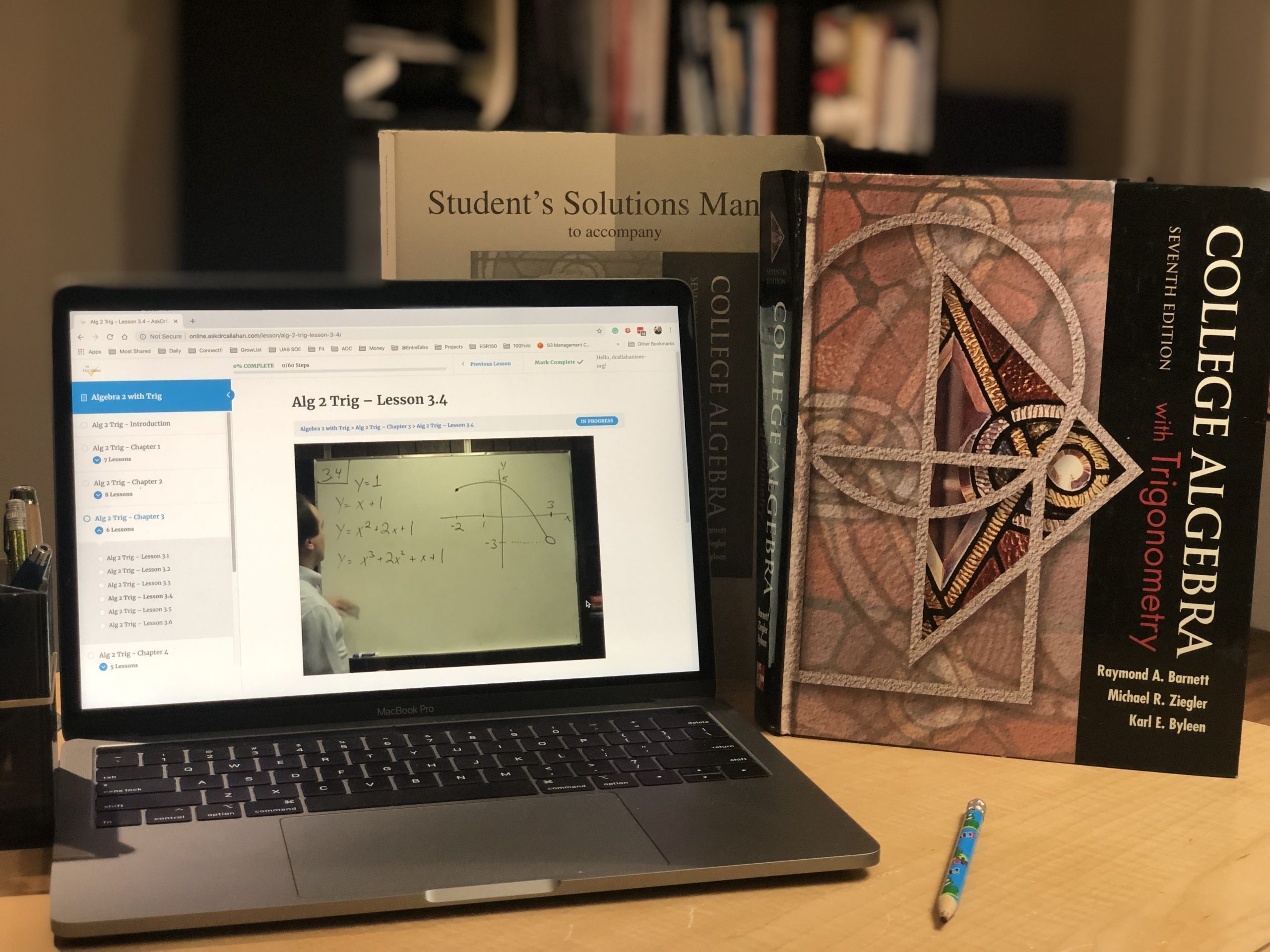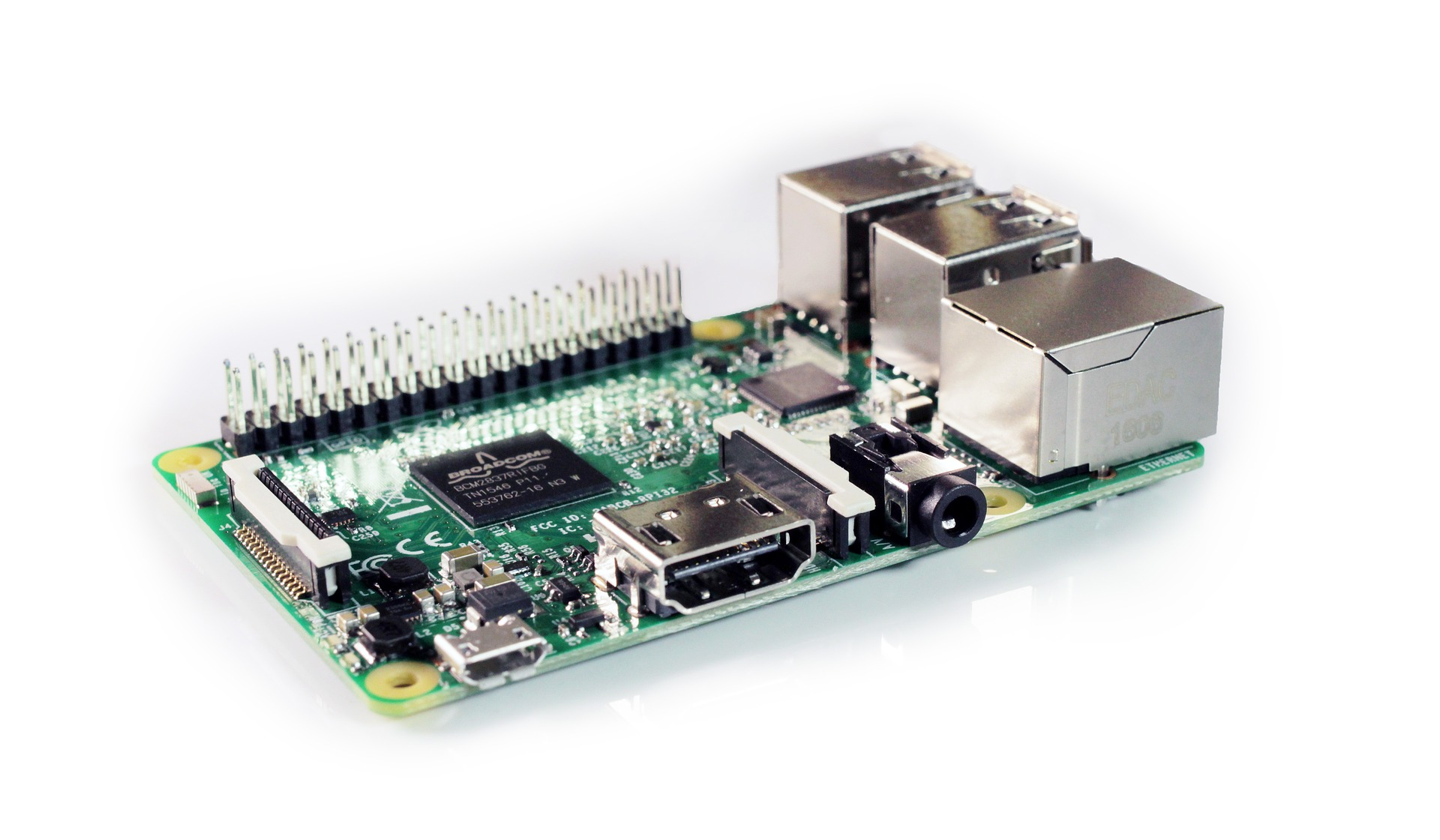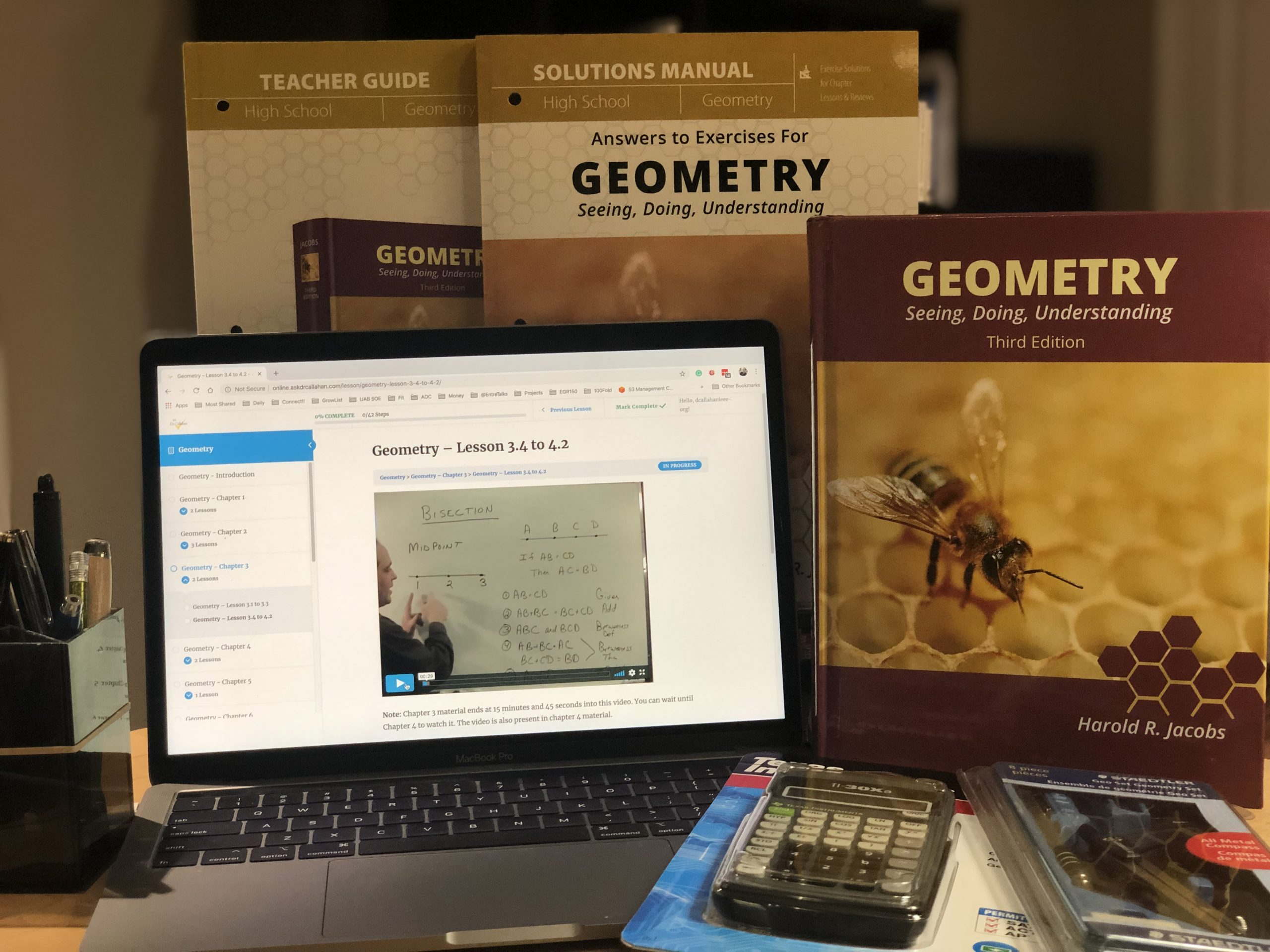Posted on

## Algebra 2 with Trig Course Description for TranscriptIf you are looking for details for a transcript for Algebra 2 with Trig,  this should do the trick.

Algebra 2 and Trigonometry builds on basic Algebra and Geometry to prepare the student for calculus or other college-level mathematics courses.

Students who complete Algebra 2 with Trig should take Calculus next. Concepts from this course show up on the ACT and the SAT.

Topics Covered in this Course:

• Algebraic Operations
• Equations and Inequalities
• Graphs and Functions
• Polynomial and Rational Functions
• Exponential and Logarithmic Functions
• Trigonometric Functions
• Trigonometric Identities and Conditional Equations
• Additional Topics and Applications in Trigonometry
Posted on

## Pre-calculus (or Algebra II with Trig) Textbook Evaluations

Look for the following material or heading in the contents.

• Review of Algebra
· Basic operations
· Factoring
· Exponents
• Equation and Inequalities
· Linear equations
· Absolute value
· Complex numbers
· Polynomials
• Graphs and Functions
· Circles
· Straight lines
· Functions
· Graphing functions
• Polynomials
· Finding zeroes of polynomials
• Rational Functions
· Graphs of rational functions
· Partial fractions
• Exponential and Logarithmic Functions
· Exponential functions
· Logarithmic functions
· Common and natural logarithms
· Exponential and logarithmic equations
• Trigonometric Functions
· Angles
· Right triangle trigonometry (Basic trig functions)
· Sine, Cosine, Tangent
· Graphing
• Analytic Trigonometry
· Trigonometric identities
· Law of Sines
· Law of Cosines
· Vectors
· Complex numbers

Optional material included in some courses.

• Systems of Equations
· Solving systems of equations
· Linear programming
• Matrices and Determinants
· Basic operations
· Square matrices
· Determinants
• Sequences and Series
· Arithmetic sequences
· Geometric sequences
· Binomial formula

Notes for use: While everything above is needed material, it is key to get through the trigonometric material – possibly leaving off systems of equations and the later material. At the Algebra II level and above college material should be used.

Posted on

## Raspberry Pi and A Robot Ferris Wheel you can build at home for less than \$100Some of the links in this article are affiliate links. We don’t carry in our shop all the materials you would need to complete this activity, so we use links to share with you the products you would want to use so that you can do this activity at home. Some of those links are affiliates and we will make a small percentage commission, at no additional cost to you, if you use our links to purchase your supplies.

Have you ever wanted to build your own Lego Ferris Wheel or Train and be able to control it like a robot? Now you can!–and BONUS it counts as a math class activity. Keep reading to find out how to do it and at the bottom I’ll show you where in your Algebra II with Trig course this activity would fall so you can schedule it in your lessons.

Posted on

## Algebra 2 with Trig Ch6.2 #45

The quick hint is this… label the bottom length x so that the entire bottom section of the RIGHT triangle is d+x.
Then  you can say
tan(ALPHA) = h / (x+d)
tan (BETA) = h/x
then solve the second for x and substitute into the first.
For details, you can see the solution in this book
Posted on

## Algebra II with Trig Ch1.4 #25

Question:

We need help on Algebra II with Trig 1.4 #25. How do we get started?

We want to reduce the problem.

The first step I take on these kind of problems is to make it easier to see. Looking at what we are given – we can see that the we have two big parts added together in the numerator

One part is the

-2x(x+4)^3

and the other part is the

-3(3-x^2)(x+4)^2

We can see that both parts have an x+4.

Often in busy problems like this, we can make things easier and replace the x+4 with k to get something like

-2xk^3 – 3(3-x^2)k^k

as the numerator. So now we can see we can factor out the k.

k^2 [-2xk – 3(2-x^2)]

We can put this back to get

(x+4)^2 [-2x(x+4) – 3(2-x^2)]  /  [ (x+4)^6]

Now we can reduce by dividing out the (x+4)^2 term to get

[-2x(x+4) – 3(2-x^2)]  /  [ (x+4)^2]

Posted on

## What course after saxon algebra 1 and algebra 2?

Question:

My daughter has taken Saxon Algebra 1 and Algebra 2 and Jacobs Geometry. I am looking for a precalculus course, but I see that yours is Algebra II with Trig. I’m wondering does that sound is that something that would make sense for someone who is taken Saxon Algebra 1 & 2 or would that be an overlap.

Algebra 2 with Trig and Precalculus are just repacked content of basically the same material. See Algebra 2 with Trig vs Precalc for more information on this.

What your daughter is likely missing is the trig part, and maybe some of the more advanced Algebra 2. Since you likely are trying to make sure she is prepared for college and not missing anything, here is what I would do:

1. Go to the publishers online Student Center (You can always find the link here at our course on the Algebra 2 with Trig Everything You Need page.)
2. Pick chapter 1, and have her take the Practice Quiz. (They are all pretty short and are graded online). If she does 80% or better, have her move on to the next chapter.
3. Do this through chapter 8 until she finds the place where she is missing a lot or is totally lost. I would expect this might be in chapter 6-8.
4. Now develop a plan to cover the content from those chapters.

Here are some options for developing a plan:

• Use the textbook we have (you can use the online version) and have her study the content and do the online quizzes as her tests. You can use our syllabus as a guide.
• You can do the above and mix in some free online videos such as Khan Academy. Here is their Algebra 2 and their Trig.
• You could buy the course and cover the content you need. (If she is weak in a lot of the chapters this might be the best option.)

Caution: If she is heading for any STEM (Science, Technology, Engineering, or Math) study in college or career, she needs to be very strong – so be careful not to skimp here. The biggest reason for kids to bail out of these career paths is weak math skills.

Posted on

## Should We Take Algebra 2 Before Geometry?Geometry is designed to be taken before Algebra 2 with Trigonometry and after Algebra 1. But this does leave some concerned that their student will forget all they learned about algebra in a year of geometry. This should not be a concern for the following reasons.

• Harold Jacobs Geometry textbook (and our videos) has Algebra reviews at the end of each chapter, so students have a smooth transition between Algebra 1 and Algebra 2 with Trig. They won’t forget the concepts.
• Geometry itself is a math course and has algebra build into the material. Your student will be solving for X often.
Posted on

## Algebra II with Trig Ch5.5 #90 (Correction to solution)

Question from Amelia:

Dear Dr. Callahan, I found a place where the answer key for even-numbered problems in Algebra II with Trig is incomplete.  In Ch 5 Section 5, problem 90 explains what a photic zone is in lakes or the ocean, and gives the formula I = Io e ^ -kd where k is the constant called the coefficient of extinction and d is the depth.  It then gives the depth where, in a certain lake, half of the surface light remains, and asks you to find both k and the depth of the photic zone.  However, the answer key only has the answer for the value of k.  It says nothing at all about the depth of the photic zone.

Thanks for pointing this out. Actually there are TWO errors in this problem – so let me resolve it

Starting fromand solving by taking the natural log (ln) of both sides we get

-0.693 = -k * 14.3

k = 0.0485 (In the Solutions to Selected Problems this is 0.485. If you tried with the other number 0.485, your answer would not make since because you get 1% of the light at about 9 feet which is more shallow than when you get half the light at 14 feet)

Now, to complete this you would need to take the k value and insert it in the equation where you are at 1% of surface light remaining.

This gives younow take the natural log (ln) to get

-4.605 = -0.0485d

d = 94.95 feet

and rounding to 3 significant digits (See page A-3 in back of book)

d = 95.0 feet

CHECK OUR WORK

Now we can check the work!

I = Io e^(-0.0485*95)
I/Io = 0.00997 or 0.01 or 1%

Hope this clears it up.

dwc

PS – Sorry for the delay – I just thought you were telling us we were missing something and did not look at it close till today!
dwc

Posted on

## Algebra II with Trig Ch1.3 #73

How do we work Algebra II with Trig Chapter 1.3 #73?Solution

a) Area after purple section cut out

A = (20 times 20) – 4(x^2) = -4x^2 + 400 = -4(x^2 – 100)  = -4(x + 10)(x – 10)

b) V = Area of bottom times height = (20-2x)(20-2x) x = (400-80x+4x^2)x = 4x^3 – 80x^2 + 400x = 4x(x^2 – 20x + 100)